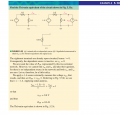# Equivalent resistance of a network with dependant sources

#### mjakov

Joined Feb 13, 2014
20
Hi there!

Does someone know why it is not possible to obtain the network equivalent resistance as seen from the terminal a-b by short circuiting the voltage sources and doing network reduction in this case? Could it have something to do with the dependent voltage source $$6I_x$$By Network Reduction:
$$R' = 4 || 6 = 2.4 \Omega$$

Applying KVL on the open circuit a-b
$$-20 + 4I_x - 6I_x + 6I_x = 0$$
$$I_x = 5 (A) V_{oc} = 30 V$$

Applying KVL on the short circuit a-b
$$I_x = 0 -20 + 4I = 0 I_{sc} = 5 A$$

By Thevenin's Theorem:
$$R' = \frac{V_{oc}}{I_{sc}} = 6 \Omega$$

#### JoyAm

Joined Aug 21, 2014
126
Well your assumption is right you cannot do that if you have a dependent source. So you have to
1) short circuit the independent source(s) but keep the dependent ones untouched
2)put an independent source of your liking
3)do what you did by yourself by thevenin's theorem.
This method will help you with more complex circuits that cannot be solved as you did here

•mjakov

#### t_n_k

Joined Mar 6, 2009
5,455
This is an unusual situation to the extent that the dependent voltage source always matches Vab. Hence it forces the left hand branch to behave as a 5A current source. Treat a current source as an open circuit when removed - hence effective 6 ohms looking into ab terminals.

•mjakov

#### MrAl

Joined Jun 17, 2014
7,849
Hi,

Dependent sources act like amplifiers, and amplifiers with feedback are a little different than just regular sources so they can not always be shorted out.

My view here is a little like tnk's view, in that it becomes clear that if we apply a voltage from a to b of Vab volts, the dependent source 6*Ix takes on the same voltage Vab and because it is in anti series, the voltage at the left of the dependent source is always zero (same as terminal b).
Because it is always zero on the left and the 20v source has zero volts on the right (terminal b is at 0v too), the 20v source and 4 ohm resistor behave as if they had nothing to do with what is happening at the input and Vab. They act as if they were current isolated with a common ground. Thus some current flows from the 20v supply, but it never affects the part of the circuit we happen to be measuring. Even the dependent source itself acts like it wasnt there, so we can remove everything except the 6 ohm resistorSo yes, the dependent source introduces a little more complexity into the calculation for impedance and that happens with any amplifier that has feedback (in many cases).

•mjakov

#### t_n_k

Joined Mar 6, 2009
5,455
It's simple. Apply a 1A source across the A-B terminal. Short the 12V source and just apply nodal analysis to find out V at C and the Rth will be Vc/1A.View attachment 72734
This doesn't seem to be a correct approach - notwithstanding the correct answer.

#### dalam

Joined Aug 9, 2014
58This doesn't seem to be a correct approach - notwithstanding the correct answer.
I have attached a snapshot of Hayt-Engineering-Circuit-Analysis-8th-Txtbk. In this example he has done it the same way. Does the presence of an independent source change it? During the analysis of Rth we short the voltage source and open the current source if they are independent.

I can solve the same problem the way it has been solved at the top but why my approach is incorrect???

Last edited:
•mjakov

#### Jony130

Joined Feb 17, 2009
5,186
This doesn't seem to be a correct approach - notwithstanding the correct answer.
I'm also interested why this approach is incorrect. And how the correct approach should looks like

#### t_n_k

Joined Mar 6, 2009
5,455
There is no problem with the method.

#### MrAl

Joined Jun 17, 2014
7,849
Hi,

You should be able to do it either way, apply a test voltage or a test current. Sometimes one works better than the other. I preferred the voltage test for this circuit because it eliminates a lot of the other components.

If we have a resistor of unknown value and apply a voltage of 1 volt and we measure 0.1 amp, V/I gives us 1/0.1=10 ohms.
If we have the same resistor and apply 1 amp and measure 10 volts, V/I again gives us 10 ohms.

Obviously there are practical issues that can come up like do we blow out the circuit or underpower it if we actually try this with a real circuit. This means the test current or voltage might have to be smaller or larger than 1v or 1 amp. For some circuits we wont see much activity until we apply enough voltage.

Last edited:
•mjakov

#### anhnha

Joined Apr 19, 2012
881
If we have a resistor of unknown value and apply a voltage of 1 volt and we measure 0.1 amp, V/R gives us 1/0.1=10 ohms.
If we have the same resistor and apply 1 amp and measure 10 volts, V/R again gives us 10 ohms.
I am sure you meant V/I not V/R.•MrAl

#### MrAl

Joined Jun 17, 2014
7,849
I am sure you meant V/I not V/R.Hi,

Yes my apologies and thanks for pointing that out. It's been corrected now. Somehow i typed V/R instead of V/I.